### Home > MC2 > Chapter 5 > Lesson 5.1.2 > Problem5-23

5-23.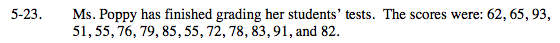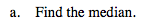Recall that the median is the middle number of an ordered set of data.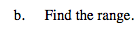The range is the interval of the values covered. To find it, take the difference between the highest and the lowest values.

42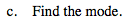Recall that mode is the number that occurs the most often within a set of data.

55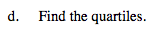Along with the median, the quartiles divide a set of data into four groups of the same size.

Lower Quartile=62
Upper Quartile=83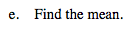Recall that the mean refers to the average of a set of data.

Explore the problem with the eTool below.
Click the link at right for the full version of the eTool: MC2 5-23 HW eTool